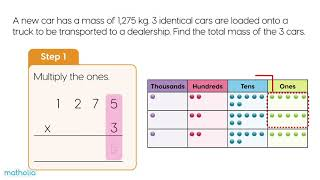## Practice and Learn

 1Multiplication by a 1-digit Number – Place Value Chart Video 2Addition and Subtraction Sheet 3Multiplying by a 1-Digit Number Sheet 4Multiplying by a 2-digit Number Sheet 5Dividing by a 2-digit Number Sheet